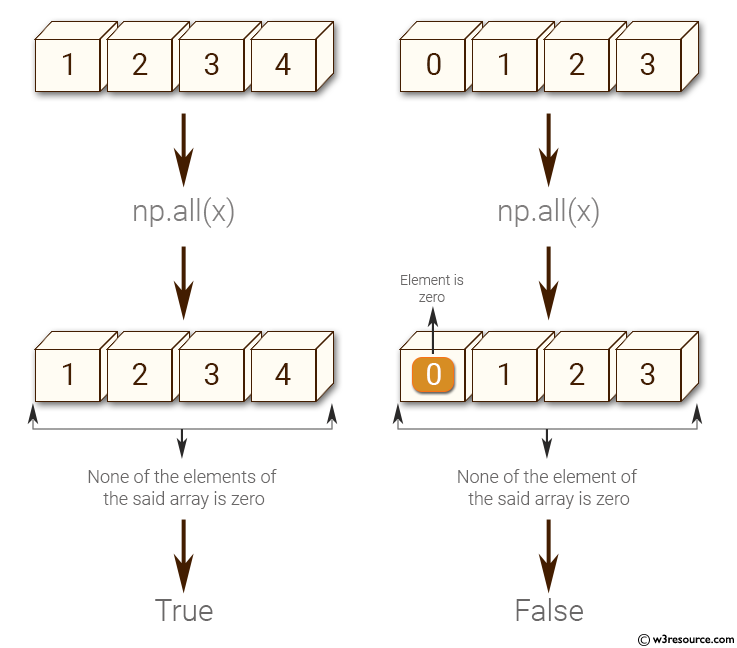﻿ NumPy: Test whether none of the elements of a given array is zero - w3resource

# NumPy: Test whether none of the elements of a given array is zero

## NumPy: Basic Exercise-3 with Solution

Write a NumPy program to test whether none of the elements of a given array is zero.

Sample Solution :

Python Code :

``````import numpy as np
x = np.array([1, 2, 3, 4])
print("Original array:")
print(x)
print("Test if none of the elements of the said array is zero:")
print(np.all(x))
x = np.array([0, 1, 2, 3])
print("Original array:")
print(x)
print("Test if none of the elements of the said array is zero:")
print(np.all(x))
``````

Sample Output:

```Original array:
[1 2 3 4]
Test if none of the elements of the said array is zero:
True
Original array:
[0 1 2 3]
Test if none of the elements of the said array is zero:
False
```

Pictorial Presentation:Python Code Editor:

Have another way to solve this solution? Contribute your code (and comments) through Disqus.

What is the difficulty level of this exercise?

﻿

Inviting useful, relevant, well-written and unique guest posts